Examples

Chapter 9 Class 12 Differential Equations
Serial order wise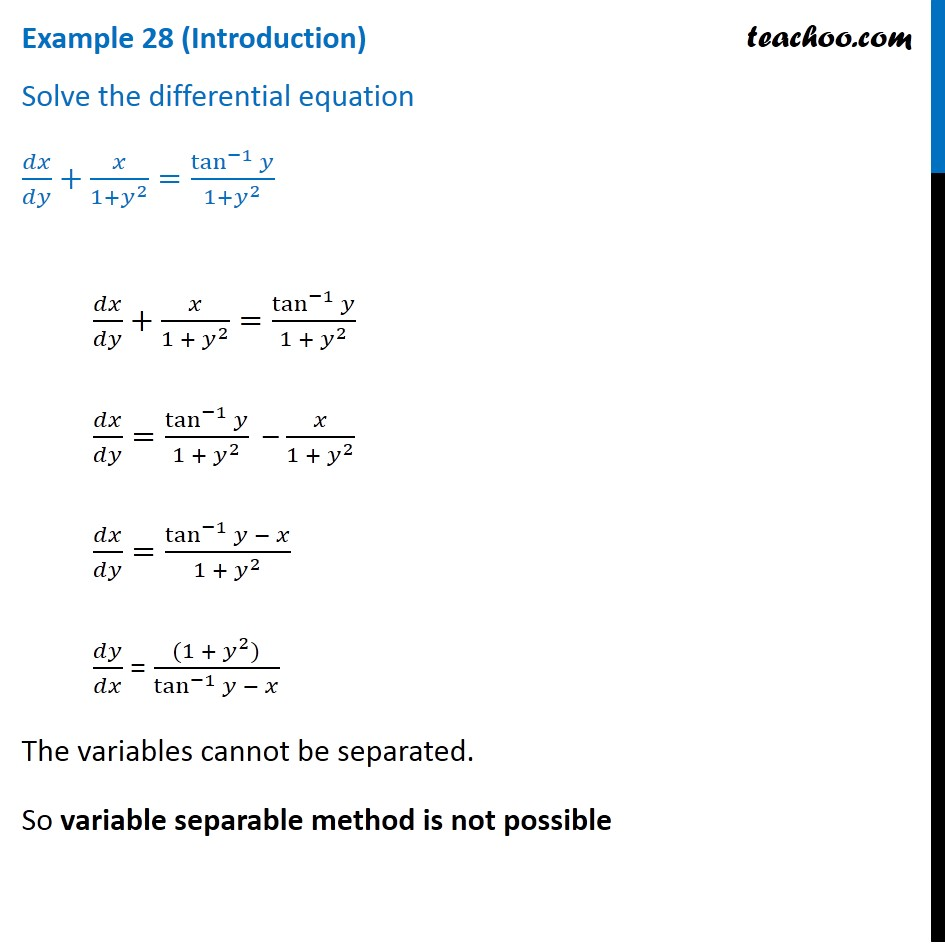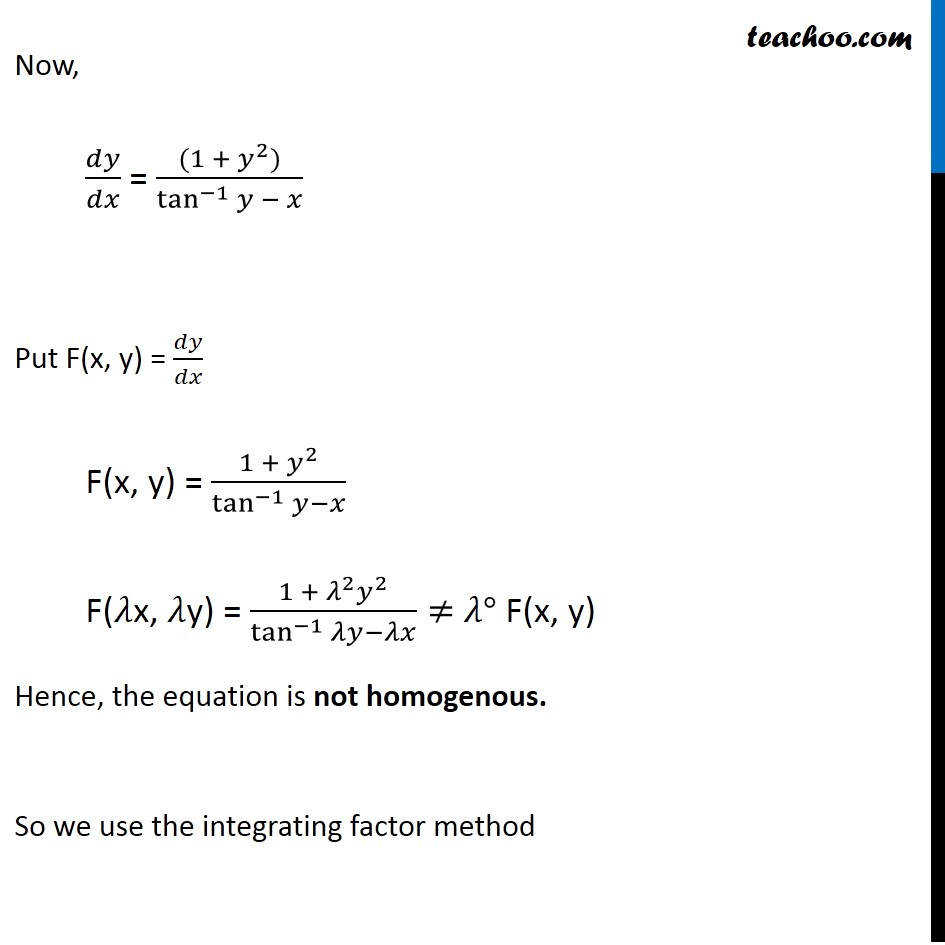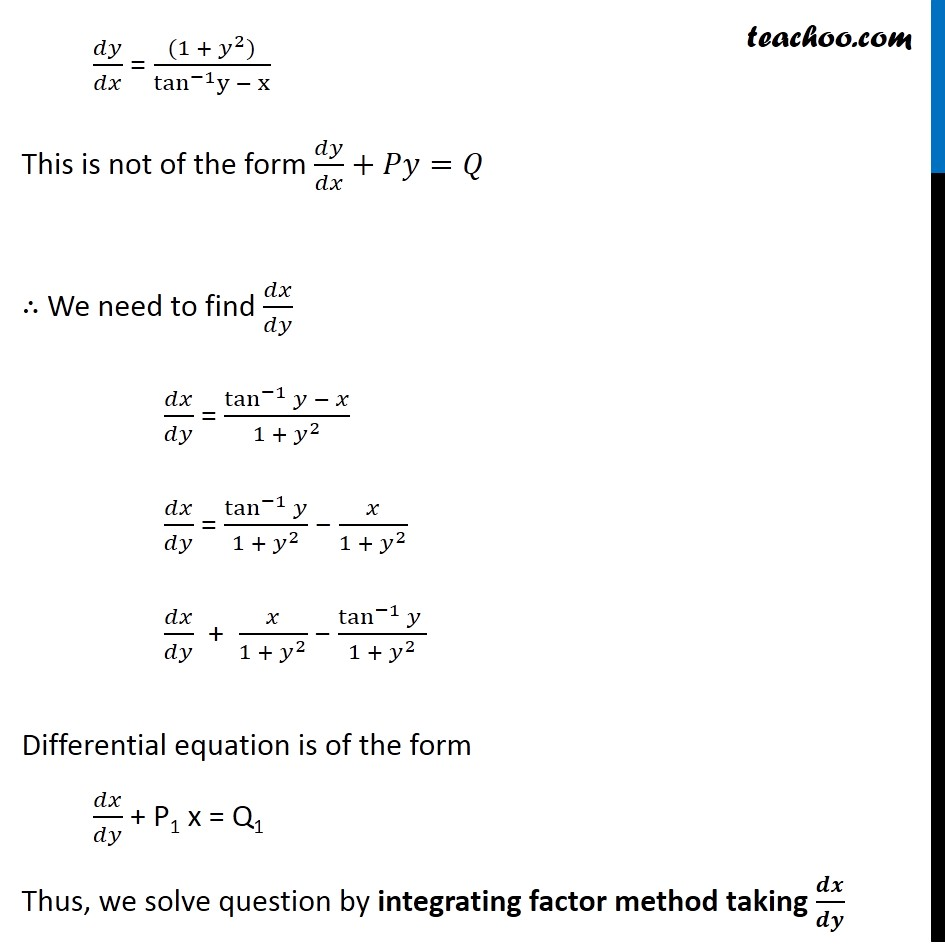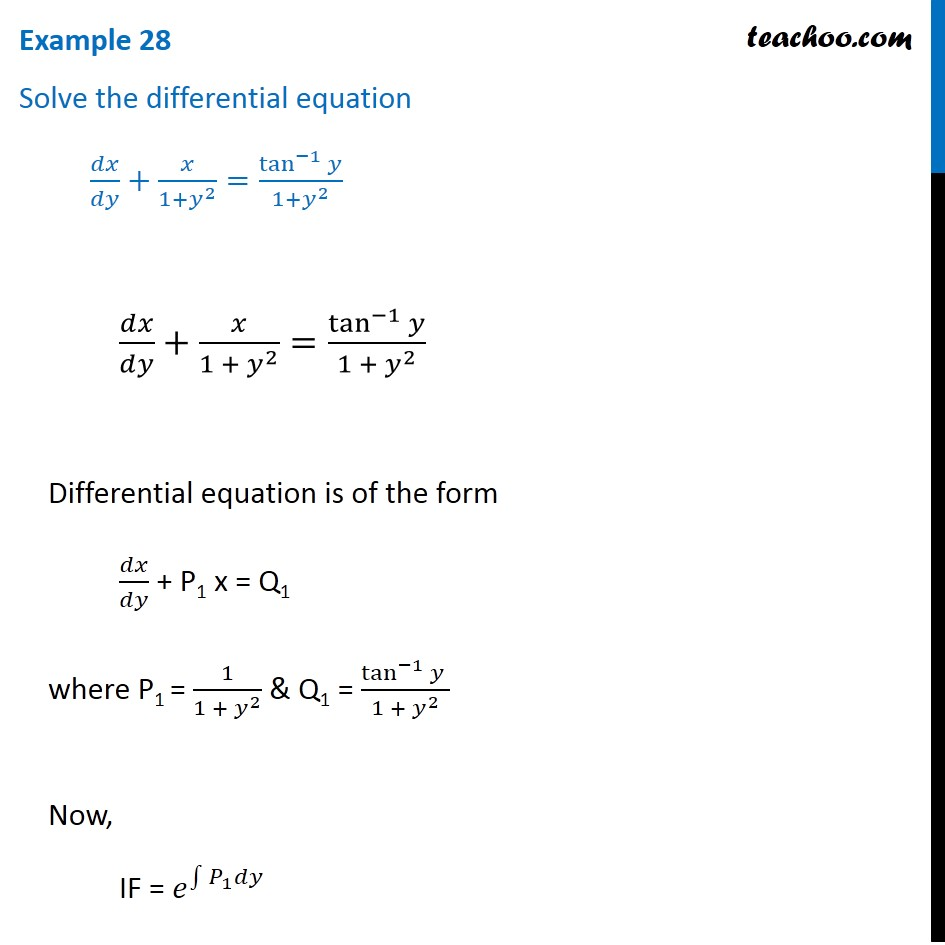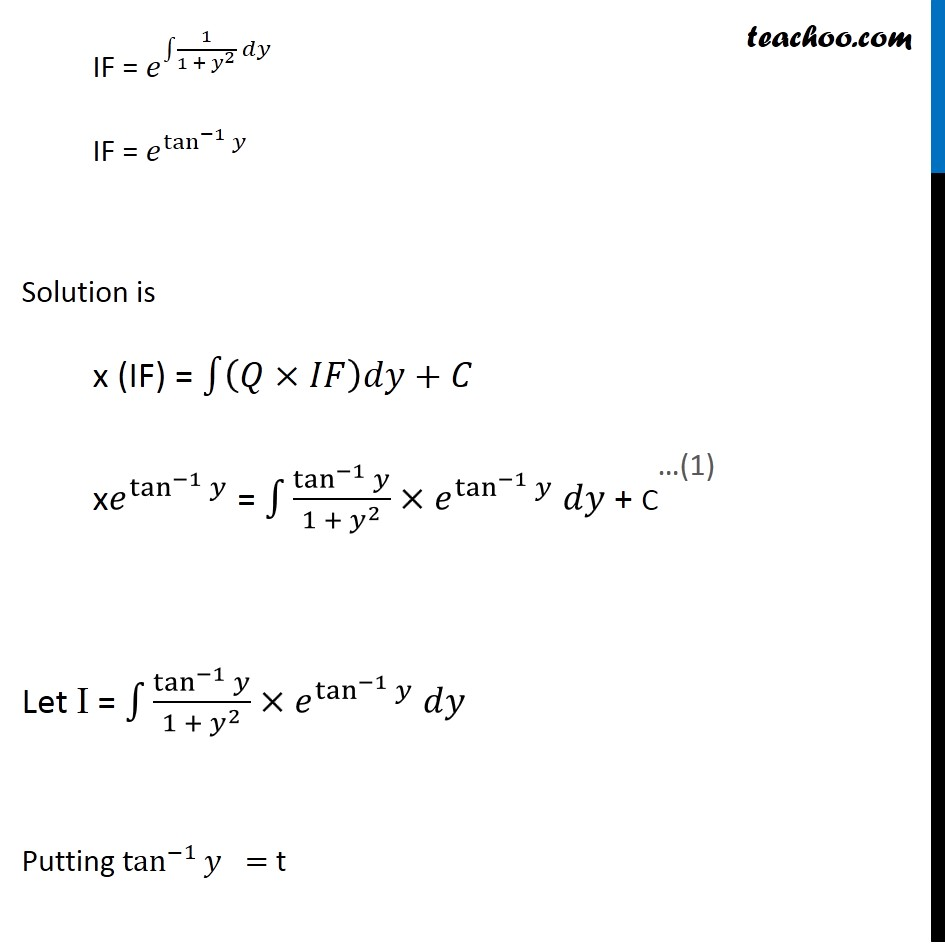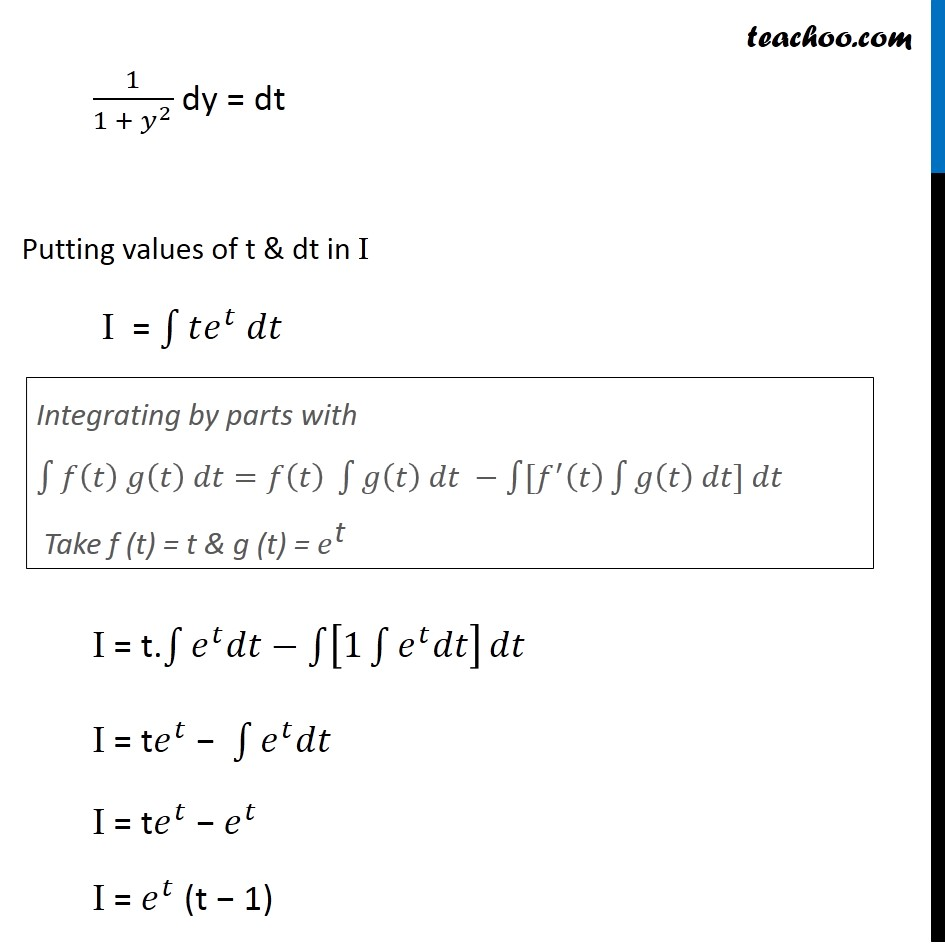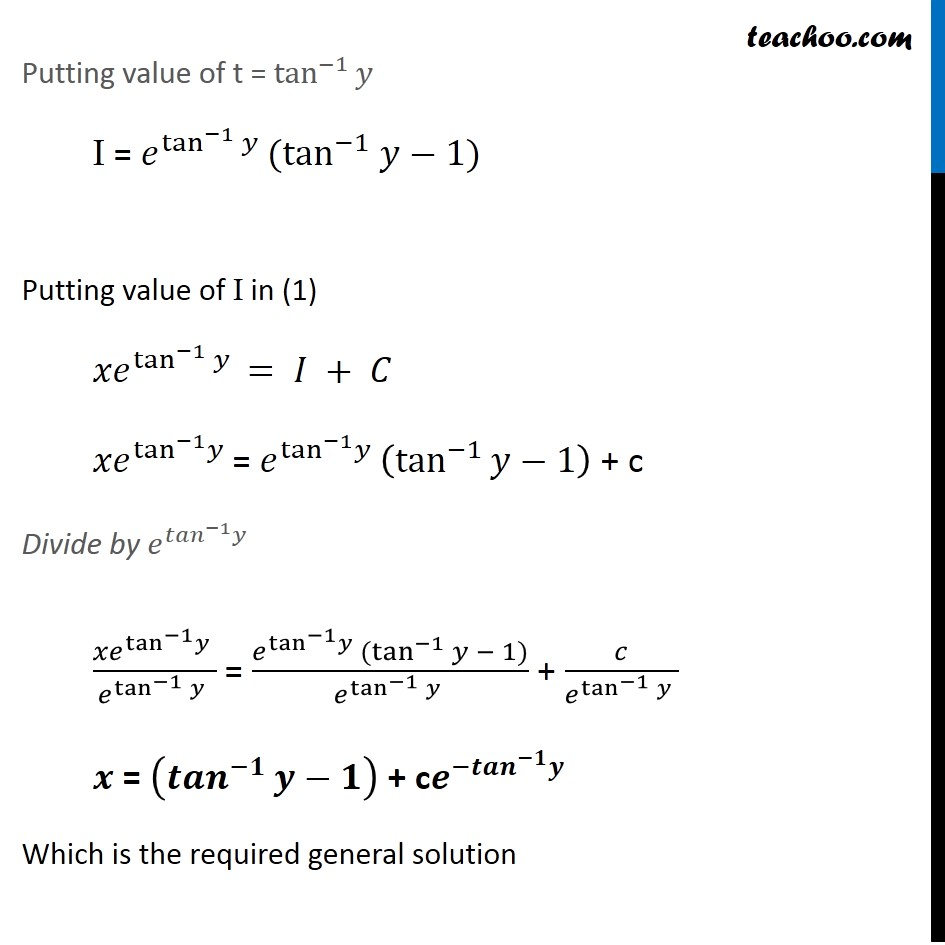Solve all your doubts with Teachoo Black (new monthly pack available now!)

### Transcript

Example 28 (Introduction) Solve the differential equation 𝑑𝑥/𝑑𝑦+𝑥/(1+𝑦^2 )=tan^(−1)⁡𝑦/(1+𝑦^2 ) 𝑑𝑥/𝑑𝑦+𝑥/(1 + 𝑦^2 )=tan^(−1)⁡𝑦/(1 + 𝑦^2 ) 𝑑𝑥/𝑑𝑦=tan^(−1)⁡𝑦/(1 + 𝑦^2 ) – 𝑥/(1 + 𝑦^2 ) 𝑑𝑥/𝑑𝑦=(tan^(−1)⁡𝑦 − 𝑥)/(1 + 𝑦^2 ) 𝑑𝑦/𝑑𝑥 = ((1 + 𝑦^2))/tan^(−1)⁡〖𝑦 − 𝑥〗 The variables cannot be separated. So variable separable method is not possible Now, 𝑑𝑦/𝑑𝑥 = ((1 + 𝑦^2))/tan^(−1)⁡〖𝑦 − 𝑥〗 Put F(x, y) = 𝑑𝑦/𝑑𝑥 F(x, y) = (1 + 𝑦^2)/(tan^(−1)⁡𝑦−𝑥) F(𝜆x, 𝜆y) = (1 + 𝜆^2 𝑦^2)/(tan^(−1)⁡𝜆𝑦−𝜆𝑥)≠ 𝜆° F(x, y) Hence, the equation is not homogenous. So we use the integrating factor method 𝑑𝑦/𝑑𝑥 = ((1 + 𝑦^2))/(tan^(−1) y − x) This is not of the form 𝑑𝑦/𝑑𝑥+𝑃𝑦=𝑄 ∴ We need to find 𝑑𝑥/𝑑𝑦 𝑑𝑥/𝑑𝑦 = (tan^(−1)⁡𝑦 − 𝑥)/(1 + 𝑦^2 ) 𝑑𝑥/𝑑𝑦 = tan^(−1)⁡𝑦/(1 + 𝑦^2 ) − 𝑥/(1 + 𝑦^2 ) 𝑑𝑥/𝑑𝑦 + 𝑥/(1 + 𝑦^2 ) − (tan^(−1)⁡𝑦 )/(1 + 𝑦^2 ) Differential equation is of the form 𝑑𝑥/𝑑𝑦 + P1 x = Q1 Thus, we solve question by integrating factor method taking 𝒅𝒙/𝒅𝒚 Example 28 Solve the differential equation 𝑑𝑥/𝑑𝑦+𝑥/(1+𝑦^2 )=tan^(−1)⁡𝑦/(1+𝑦^2 ) 𝑑𝑥/𝑑𝑦+𝑥/(1 + 𝑦^2 )=tan^(−1)⁡𝑦/(1 + 𝑦^2 ) Differential equation is of the form 𝑑𝑥/𝑑𝑦 + P1 x = Q1 where P1 = 1/(1 + 𝑦^2 ) & Q1 = (tan^(−1)⁡𝑦 )/(1 + 𝑦^2 ) Now, IF = 𝑒^∫1▒〖𝑃_1 𝑑𝑦〗 IF = 𝑒^∫1▒〖1/(1 + 𝑦^2 ) 𝑑𝑦〗 IF = 𝑒^tan^(−1)⁡𝑦 Solution is x (IF) = ∫1▒〖(𝑄×𝐼𝐹)𝑑𝑦+𝐶〗 x𝑒^tan^(−1)⁡𝑦 = ∫1▒〖tan^(−1)⁡𝑦/(1 + 𝑦^2 )×𝑒^tan^(−1)⁡𝑦 𝑑𝑦〗 + C Let I = ∫1▒〖tan^(−1)⁡𝑦/(1 + 𝑦^2 )×𝑒^tan^(−1)⁡𝑦 𝑑𝑦〗 Putting tan^(−1)⁡〖𝑦 〗= t 1/(1 + 𝑦^2 ) dy = dt Putting values of t & dt in I I = ∫1▒〖𝑡𝑒^𝑡 𝑑𝑡〗 I = t.∫1▒〖𝑒^𝑡 𝑑𝑡−∫1▒[1∫1▒〖𝑒^𝑡 𝑑𝑡〗] 𝑑𝑡〗 I = t𝑒^𝑡 − ∫1▒〖𝑒^𝑡 𝑑𝑡〗 I = t𝑒^𝑡 − 𝑒^𝑡 I = 𝑒^𝑡 (t − 1) Putting value of t = tan^(−1)⁡𝑦 I = 𝑒^(tan^(−1)⁡𝑦 ) (tan^(−1) 𝑦−1) Putting value of I in (1) 〖𝑥𝑒〗^(tan^(−1)⁡𝑦 ) = 𝐼 + 𝐶 Integrating by parts with ∫1▒〖𝑓(𝑡) 𝑔(𝑡) 𝑑𝑡=𝑓(𝑡) ∫1▒〖𝑔(𝑡) 𝑑𝑡 −∫1▒〖[𝑓^′ (𝑡) ∫1▒〖𝑔(𝑡) 𝑑𝑡] 𝑑𝑡〗〗〗〗 Take f (t) = t & g (t) = 𝑒^"t" Divide by 𝑒^(〖𝑡𝑎𝑛〗^(−1) 𝑦 ) 〖𝑥𝑒〗^(tan^(−1) 𝑦 )/𝑒^tan^(−1)⁡〖𝑦 〗 = (𝑒^(tan^(−1) 𝑦) (tan^(−1)⁡𝑦 − 1))/𝑒^tan^(−1)⁡〖𝑦 〗 + 𝑐/𝑒^tan^(−1)⁡〖𝑦 〗 𝒙 = (〖𝒕𝒂𝒏〗^(−𝟏)⁡𝒚−𝟏) + c𝒆^(−〖𝒕𝒂𝒏〗^(−𝟏) 𝒚 ) Which is the required general solution